# pig tutorial - apache pig tutorial - Apache Pig - Join Operator - pig latin - apache pig - pig hadoop

## What is Join?

• JOIN keyword is used to combine rows from two or more logs, based on a common fields .
• Left join returns all logs from left log(table) and matching logs from the right log.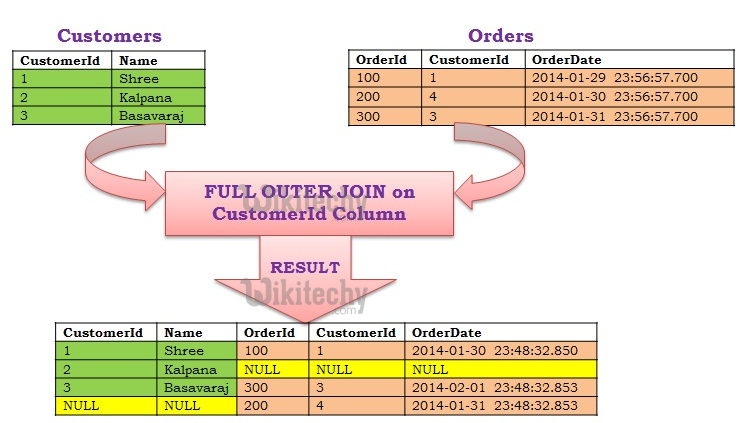Learn Apache Pig - Apache Pig tutorial - customer id column - Apache Pig examples - Apache Pig programs

## Pig Operations - Joining

• JOIN joins two or more sets
•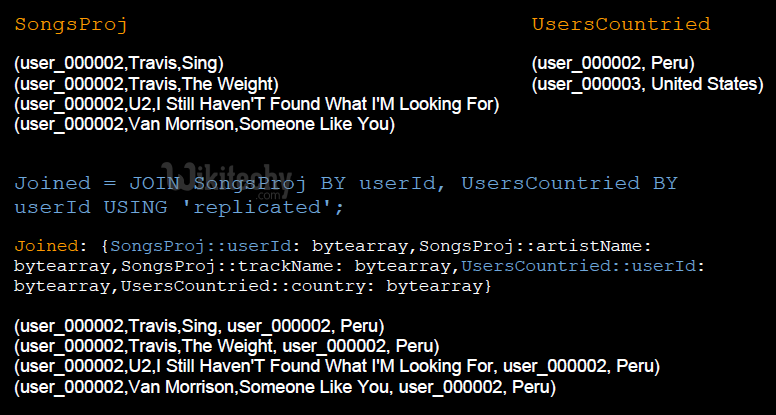• Good build-in support for joins
• Many different join implementations
• Left, right and full outer joins are supported
• Joining on multiple keys is supported
• Merge Join
• sets are pre-sorted by the join key
• Merge-Sparse Join (since Pig 0.10.0)
• sets are pre-sorted and one set has few ( < 1% of its total) matching keys
• Replicated Join
• one set is very large, while other sets are small enough to fit into memory
• Skewed Join
• when a large number of records for some values of the join key is expected
• Regular Join
• (inner) JOIN instruction:
• Our classic database operator for relations!• (left) JOIN instruction:
• Our classic database operator for relations!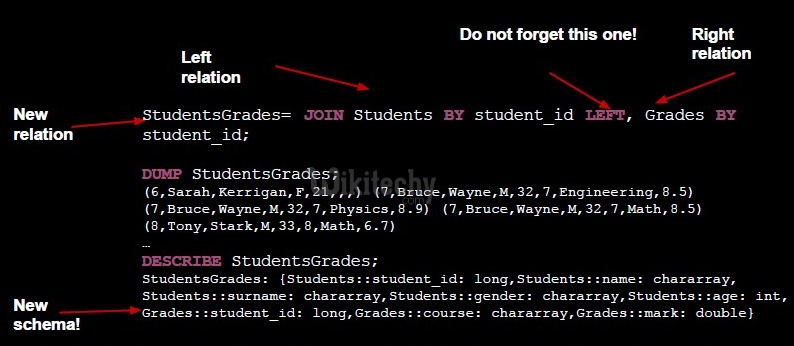## How to use join operator in Apache Pig ?

• The JOIN operator is used to combine records from two or more relations. While performing a join operation, we declare one (or a group of) tuple(s) from each relation, as keys. When these keys match, the two particular tuples are matched, else the records are dropped.
• Self-join
• Inner-join
• Outer-join − left join, right join, and full join

wikitechy_customers.txt

orders.txt

And these two files into Pig with the relations wikitechy_customers and orders as given below.

• To perform various Join operations on these two relations.

## Self - join

• Self-join is used to join a table with itself as if the table were two relations, temporarily renaming at least one relation.
• Usually, in Apache Pig, to perform self-join, we will load the same data multiple times, under different aliases (names).
• Therefore load the contents of the file wikitechy_customers.txt as two tables as given below.

## Syntax

• Given below is the syntax of performing self-join operation using the JOIN operator.

## Example

• Let us perform self-join operation on the relation customers, by joining the two relations customers1 and customers2 as given below.

## Verification

• Now verify the relation customers3 using the DUMP operator as given below.

## Output

• The following output, displaying the contents of the relation customers

## Inner Join

• Inner Join is used quite frequently; it is also referred to as equijoin. An inner join returns rows when there is a match in both tables.
• It creates a new relation by combining column values of two relations (say A and B) based upon the join-predicate.
• The query compares each row of A with each row of B to find all pairs of rows which satisfy the join-predicate.
• When the join-predicate is satisfied, the column values for each matched pair of rows of A and B are combined into a result row.Learn Apache Pig - Apache Pig tutorial - inner join - Apache Pig examples - Apache Pig programs

## Syntax

• Here is the syntax of performing inner join operation using the JOIN operator.

## Example

• Let us perform inner join operation on the two relations wikitechy_customers and orders as given below.

## Verification

• Verify the relation wikitechy_coustomer_orders using the DUMP operator as given below.

## Output

• The following output that will the contents of the relation named wikitechy_coustomer_orders.

## Note

• Outer Join: Unlike inner join, outer join returns all the rows from at least one of the relations. An outer join operation is carried out in three ways ,
• Left outer join
• Right outer join
• Full outer join

## Left Outer Join

• The left outer Join operation returns all rows from the left table, even if there are no matches in the right relation.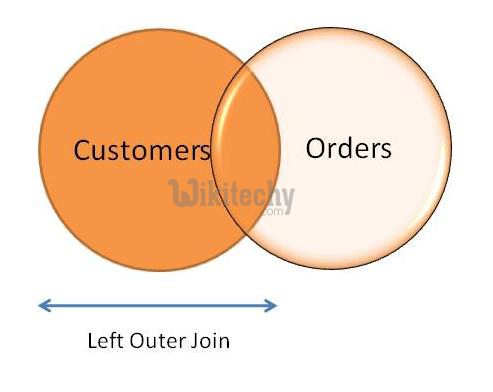Learn Apache Pig - Apache Pig tutorial - left outer join - Apache Pig examples - Apache Pig programs

## Syntax

• Let us perform left outer join operation using the JOIN operator.

## Example

• Let us perform left outer join operation on the two relations customers and orders as given below.

## Verification

• Verify the relation outer_left using the DUMP operator as given below.

## Output

• The following output, displaying the contents of the relation outer_left.

## Right Outer Join

• The right outer join operation returns all rows from the right table, even if there are no matches in the left table.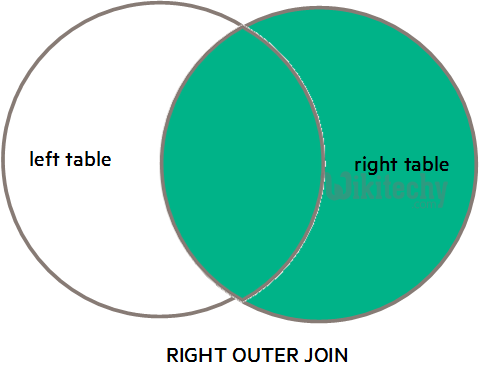Learn Apache Pig - Apache Pig tutorial - right outer join - Apache Pig examples - Apache Pig programs

## Syntax

• Given below is the syntax of performing right outer join operation using the JOIN operator.

## Example

• Let us perform right outer join operation on the two relations wikitechy_customers and orders as given below.

## Verification

• Verify the relation outer_right using the DUMP operator as given below.

## Output

• The following output, displaying the contents of the relation outer_right.
• The full outer join operation returns rows when there is a match in one of the relationsLearn Apache Pig - Apache Pig tutorial - full join new - Apache Pig examples - Apache Pig programs

## Syntax

• Given below is the syntax of performing full outer join using the JOIN operator.

## Example

• Let us perform full outer join operation on the two relations wikitechy_customers and orders as given below.

## Verification

• Verify the relation outer_full using the DUMP operator as given below.

## Output

• The following output, displaying the contents of the relation outer_full.

## Using Multiple Keys

• Let us perform JOIN operation using multiple keys are given below.

## Syntax

• Here is how you can perform a JOIN operation on two tables using multiple keys.
• Ensure that we have two files namely wikitechy_employee.txt and wikitechy_employee_contact.txt in the /pig_data/ directory of HDFS as given below.

wikitechy_employee.txt

wikitechy_employee_contact.txt

• And we have loaded these two files into Pig with relations wikitechy_employee and wikitechy_employee_contact as given below.
• Here join the contents of these two relations using the JOIN operator as given below.

## Verification

• To verify the relation emp using the DUMP operator as shown below.

## Output

• The following output, displaying the contents of the relation named emp as given below.